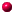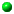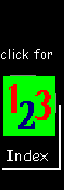### Multiplication

The tables were the communal rhythm of our classroom
The poetry of our together recitation
The binding of our togetherness
Our educationand nought times anything is nought All together with great enthusiasm: Reciting the obvious once one is one once two is two once three is three once four is four once five is five once six is six once seven is seven once eight is eight once nine is nine once ten is ten once eleven is eleven once twelve is twelve
 2 x 2 = 4 2 x 3 = 6 2 x 4 = 8 2 x 5 = 10 2 x 6 = 12 2 x 7 = 14 2 x 8 = 16 2 x 9 = 14 2 x 10 = 20 2 x 11 = 22 2 x 12 = 24 Doubling it two twos are four two threes are six two fours are eight two fives are ten two sixes are twelve two sevens are fourteen two eights are sixteen two nines are eighteen two tens are twenty two elevens are twenty-two two twelves are twenty-four
 three in the womb at once is triplets 3 x 2 = 6 3 x 3 = 9 3 x 4 = 12 3 x 5 = 15 3 x 6 = 18 3 x 7 = 21 3 x 8 = 24 3 x 9 = 27 3 x 10 = 30 3 x 11 = 33 3 x 12 = 36 Treble it three ones are three three twos are six three threes are nine three fours are twelve three fives are fifteen three sixes are eighteen three sevens are twenty-one three eights are twenty-four three nines are twenty-seven three tens are thirty three elevens are thirty-three three twelves are thirty-six
 four in the womb at once is quads 4 x 2 = 8 4 x 3 = 12 4 x 4 = 16 4 x 5 = 20 4 x 6 = 24 4 x 7 = 28 4 x 8 = 32 4 x 9 = 36 4 x 10 = 40 4 x 11 = 44 4 x 12 = 48 Quadruple it four ones are four four twos are eight four threes are twelve four fours are sixteen four fives are twenty four sixes are twenty four four sevens are twenty eight four eights are thirty two four nines are thirty six four tens are forty four elevens are forty four four twelves are forty eight
 cinquefoil has five lobed leaves 5 x 2 = 10 5 x 3 = 15 5 x 4 = 20 5 x 5 = 25 5 x 6 = 30 5 x 7 = 35 5 x 8 = 40 5 x 9 = 45 5 x 10 = 50 5 x 11 = 55 5 x 12 = 60 Cinqueruple it five ones are five five twos are ten five threes are fifteen five fours are twenty five fives are twenty five five sixes are thirty five sevens are thirty five five eights are forty five nines are forty five five tens are fifty five elevens are fifty five five twelves are sixty
 sexy six 6 x 2 = 12 6 x 3 = 18 6 x 4 = 24 6 x 5 = 30 6 x 6 = 36 6 x 7 = 42 6 x 8 = 48 6 x 9 = 54 6 x 10 = 60 6 x 11 = 66 6 x 12 = 72 Sexruple it six ones are six six twos are twelve six threes are eighteen six fours are twenty four six fives are thirty six sixes are thirty six six sevens are forty two six eights are forty eight six nines are fifty four six tens are sixty six elevens are sixty six six twelves are seventy two
 September once was seventh month 7 x 2 = 14 7 x 3 = 21 7 x 4 = 28 7 x 5 = 35 7 x 6 = 42 7 X 7 = 49 7 x 8 = 56 7 x 9 = 63 7 x 10 = 70 7 x 11 = 77 7 x 12 = 84 Septruple it seven ones are seven seven twos are fourteen seven threes are twenty one seven fours are twenty eight seven fives are thirty five seven sixes are forty two seven sevens are forty nine seven eights are fifty six seven nines are sixty three seven tens are seventy seven elevens are seventy seven seven twelves are eighty four
 an octopus has eight 8 x 2 = 16 8 x 3 = 24 8 x 4 = 32 8 x 5 = 40 8 x 6 = 48 8 x 7 = 56 8 x 8 = 64 8 x 9 = 72 8 x 10 = 80 8 x 11 = 88 8 x 12 = 96 Octruple it eight ones are eight eight twos are sixteen eight threes are twenty four eight fours are thirty two eight fives are forty eight sixes are forty eight eight sevens are fifty six eight eights are sixty four eight nines are seventy two eight tens are eighty eight elevens are eighty eight eight twelves are ninety six
 nine rhyme 9 x 2 = 18 9 x 3 = 27 9 x 4 = 36 9 x 5 = 45 9 x 6 = 54 9 x 7 = 63 9 x 8 = 72 9 x 9 = 81 9 x 10 = 90 9 x 11 = 99 9 x 12 = 108 Nunruple it nine ones are nine nine twos are eighteen nine threes are twenty seven nine fours are thirty six nine fives are forty five nine sixes are fifty four nine sevens are sixty three nine eights are seventy two nine nines are eighty one nine tens are ninety nine elevens are ninety nine nine twelves are one hundred and eight
 December once was tenth month 10 x 2 = 20 10 x 3 = 30 10 x 4 = 40 10 x 5 = 50 10 x 6 = 60 10 x 7 = 70 10 x 8 = 80 10 x 9 = 90 10 x 10 = 100 10 x 11 = 110 10 x 12 = 120 Decuple it ten ones are ten ten twos are twenty ten threes are thirty ten fours are forty ten fives are fifty ten sixes are sixty ten sevens are seventy ten eights are eighty ten nines are ninety ten tens are a one hundred ten elevens are one hundred and ten ten twelves are one hundred and twenty
 Undecagons have eleven sides 11 x 2 = 22 11 x 3 = 33 11 X 4 = 44 11 X 5 = 55 11 X 6 = 66 11 X 7 = 77 11 X 8 = 88 can you guess 11 x 9? 11 x 10 = 110 11 x 11 = 121 11 x 12 = 132 Undecuple it eleven ones are eleven eleven twos are twenty two eleven threes are thirty three eleven fours are forty four eleven fives are fifty five eleven sixes are sixty six eleven sevens are seventy seven eleven eights are eighty eight eleven nines are ninety nine eleven tens are a one hundred and ten eleven elevens are one hundred and twenty one eleven twelves are one hundred and thirty two
 A duodecad has twelve years 12 x 2 = 24 12 x 3 = 36 12 x 4 = 48 12 x 5 = 60 12 x 6 = 72 12 x 7 = 84 12 x 8 = 96 12 x 9 = 108 12 x 10 = 120 12 x 11 = 132 12 x 12 = 144 Duodecuple it twelve ones are twelve twelve twos are twenty four twelve threes are thirty six twelve fours are forty eight twelve fives are sixty twelve sixes are seventy two twelve sevens are eighty four twelve eights are ninety six twelve nines are one hundred and eight twelve tens are a one hundred and twenty twelve elevens are one hundred and thirty two twelve twelves are one hundred and forty fourTop of PageSilver shadowsNaked SnailsCosyHungeringSnakes and LaddersCuccu Songs and CarolingEurhythmy - Therapeutic Rhythms

If you think that I you have caught within a maze: Be amazed. You too could be a poem and escape. Whatever life's tricks, and, wherever you roam, you are never far from home

Andrew Roberts likes to hear from users:
To contact him, please use the Communication Form